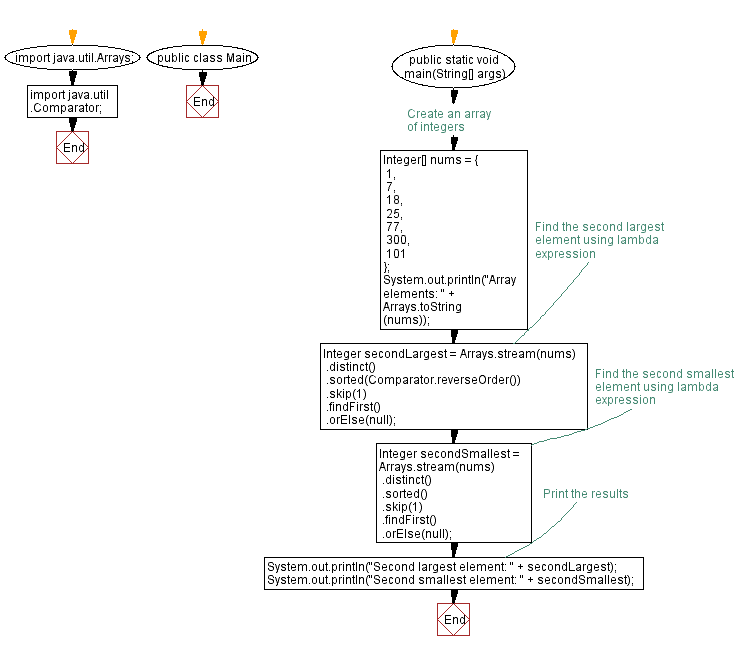# Java Lambda Expression: Find second largest and smallest element in an Array

## Java Lambda Program: Exercise-19 with Solution

Write a Java program to implement a lambda expression to find the second largest and smallest element in an array.

Sample Solution:

Java Code:

``````import java.util.Arrays;
import java.util.Comparator;

public class Main {
public static void main(String[] args) {
// Create an array of integers
Integer[] nums = {
1,
7,
18,
25,
77,
300,
101
};
System.out.println("Array elements: " + Arrays.toString(nums));
// Find the second largest element using lambda expression
Integer secondLargest = Arrays.stream(nums)
.distinct()
.sorted(Comparator.reverseOrder())
.skip(1)
.findFirst()
.orElse(null);

// Find the second smallest element using lambda expression
Integer secondSmallest = Arrays.stream(nums)
.distinct()
.sorted()
.skip(1)
.findFirst()
.orElse(null);

// Print the results
System.out.println("Second largest element: " + secondLargest);
System.out.println("Second smallest element: " + secondSmallest);
}
}
``````

Sample Output:

```Array elements: [1, 7, 18, 25, 77, 300, 101]
Second largest element: 101
Second smallest element: 7
```

Explanation:

In the above exercise,

• Import the necessary classes Arrays and Comparator.
• In the main method, we create an array of integers named numbers.

To find the second largest element:

• Use the Arrays.stream method to convert the array into a stream of integers.
• Apply the distinct operation to remove any duplicate elements.
• Sort the elements in reverse order using Comparator.reverseOrder().
• Skip the first element (the largest) using skip(1).
• Find the first element remaining, which will be the second largest, using findFirst().
• If the stream is empty, we return null using orElse(null).
• The result is stored in the variable secondLargest.

To find the second smallest element:

• Follow a similar process as above, but this time we sort the elements in ascending order using the default comparator.
• The result is stored in the variable secondSmallest.

Finally, we print the second largest and second smallest elements to the console.

Flowchart:Live Demo:

Java Code Editor:

Improve this sample solution and post your code through Disqus

Java Lambda Exercises Previous: Java program to find length of longest and smallest string using lambda expression.
Java Lambda Exercises Next: Sort list of objects with lambda expression.

What is the difficulty level of this exercise?

Test your Programming skills with w3resource's quiz.

﻿